#Application to Differential EquationsConsider the linear differential equation with constant coefficients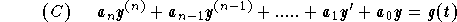under the initial conditions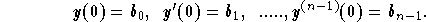The Laplace transform directly gives the solution without going through the general solution. The steps to follow are:

(1)
Evaluate the Laplace transform of the two sides of the equation (C);
(2)
Use Property 14 (see Table of Laplace Transforms)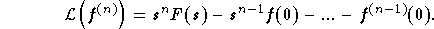;

(3)
After algebraic manipulation, write down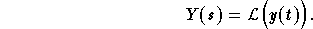;

(4)
Make use of the properties of the inverse Laplace transform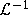, to find the solution y(t).

Example: Find the solution of the IVP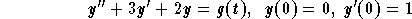,

where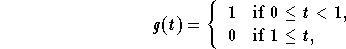.

Solution: Let us follow these steps:

(1)
We have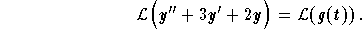;

(2)
Using properties of Laplace transform, we get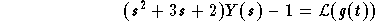,

where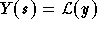. Since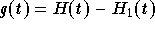, we get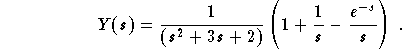;

(3)
Inverse Laplace:

Using partial decomposition technique we get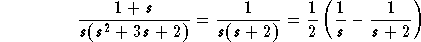,

which implies (see Table of Laplace Transforms)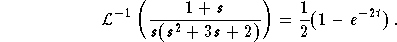Since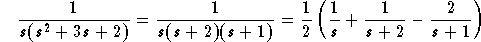,

which gives (see Table of Laplace Transforms)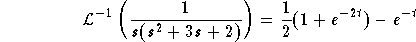,

and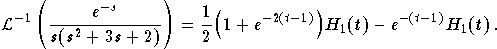Hence,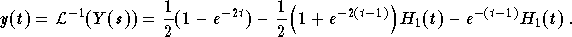If you would like more practice, click on Example.[Differential Equations] [First Order D.E.] [Second Order D.E.]
[Geometry] [Algebra] [Trigonometry ]
[Calculus] [Complex Variables] [Matrix Algebra]S.O.S MATHematics home page

Do you need more help? Please post your question on our S.O.S. Mathematics CyberBoard.Author: Mohamed Amine Khamsi